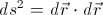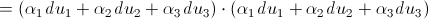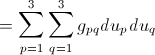## Pages

### 3. Arc Length

Let us consider a curvilinear co-ordinate system $(u_{1},u_{2},...,u_{n})$. Let $\vec{r}$ be any position vector of the curvilinear system. We then have, that $\vec{r}=\vec{r}(u_{1},u_{2},...,u_{n})$. Now, from the vector differential calculus we have the differential element of $d(\vec{r})$ as equal to the following;
(Nb: we are considering for the case of $n=3$);
Here $h_{1},h_{2},h_{3}$ is﻿ the scale factor. $e_{1},e_{2},e_{3}$ are the direction vector of the unit tangent vectors of the respective coordinate. $"h_{n}"$ is simply the magnitude of the tangent vector i.e.
Now the differential elements of the arc length in general curvilinear system is defined to be,More elegantly,
Here, we have made use of the Einstein's summation convention. This is the equation of the arc length of the curvilinear coordinate system. Here,

This $g_{pq}$ is called the metric coefficient of the concerned cuvilinear coordinates system. The value of $g$'s are different in different curvilinear coordinate system. It can be easily be seen that this $g$ is a matrix of the order ($p\times q$).

Some examples along with notes as above can be found here.